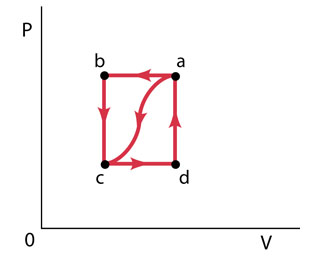#### Didn’t find what you are looking for?

Question# When a gas is taken from a to c along the curved path in the figure (Figure 1) , the work done by the gas is W = -40 J and the heat added to the gas i

Other
ANSWEREDWhen a gas is taken from a to c along the curved path in the figure (Figure 1) , the work done by the gas is W = -40 J and the heat added to the gas is Q = -140 J . Along path abc, the work done by the gas is W = -50 J . (That is, 50 J of work is done on the gas.)
I keep on missing Part D. The answer for part D is not -150,150,-155,108,105( was close but it said not quite check calculations)
Part A
What is Q for path abc?
Express your answer to two significant figures and include the appropriate units.
Part B
f Pc=1/2Pb, what is W for path cda?
Express your answer to two significant figures and include the appropriate units.
Part C
What is Q for path cda?
Express your answer to two significant figures and include the appropriate units.
Part D
What is Ua?Uc?
Express your answer to two significant figures and include the appropriate units.
Part E
If Ud?Uc=42J, what is Q for path da?
Express your answer to two significant figures and include the appropriate units.2021-05-06
Part D:
For the variation of the internal energy in the path cda we have that
$$\displaystyle\triangle{U}_{{{c}{a}}}=\triangle{U}_{{{c}{d}}}+\triangle{U}_{{{d}{a}}}$$
$$\displaystyle\triangle{U}_{{{c}{a}}}={\left({Q}_{{{c}{d}}}-{W}_{{{c}{d}}}\right)}+{\left({Q}_{{{d}{a}}}-{W}_{{{d}{a}}}\right)}$$
the path da is a constant volume, so the work in this path is zero
$$\displaystyle\triangle{U}_{{{c}{a}}}={\left({Q}_{{{c}{d}}}-{W}_{{{c}{d}}}\right)}+{\left({Q}_{{{d}{a}}}-{0}\right)}$$
$$\displaystyle\triangle{U}_{{{c}{a}}}={Q}_{{{c}{d}}}+{Q}_{{{d}{a}}}-{W}_{{{c}{d}}}$$
$$\displaystyle\triangle{U}_{{{c}{a}}}={Q}_{{{c}{a}}}-{W}_{{{c}{d}}}$$
Here we have, form the parts B and C, the $$\displaystyle{Q}_{{{c}{a}}}={130}{J}$$ and the $$\displaystyle{W}_{{{c}{d}}}={25}\ {J}$$, so
$$\displaystyle\triangle{U}_{{{c}{a}}}={105}{J}$$
An Answer that you already said is incorrect. But there is a "trick", the significant figures. You need an answer with two significant figures but that has three. So, rounding, the answer is
$$\displaystyle\triangle{U}_{{{c}{a}}}={110}{J}$$
Part E:
Since the path dc is at constant volume
$$\displaystyle\triangle{U}_{{{c}{d}}}={Q}_{{{c}{d}}}$$
And like we have $$\displaystyle{Q}_{{{c}{d}{a}}}$$
$$\displaystyle{Q}_{{{c}{d}{a}}}={Q}_{{{c}{d}}}+{Q}_{{{d}{a}}}$$
$$\displaystyle{Q}_{{{d}{a}}}={Q}_{{{c}{d}{a}}}-{Q}_{{{c}{d}}}$$
$$\displaystyle{Q}_{{{d}{a}}}={130}{J}-{42}{J}$$
$$\displaystyle{Q}_{{{d}{a}}}={88}{J}$$